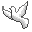Community Discussion list Contact Andrius Kulikauskas m a t h 4 w i s d o m @ g m a i l . c o m +370 607 27 665 Eičiūnų km, Alytaus raj, LithuaniaMy original work is in the Public Domain for you to use in your best judgement. Thank you for your support! Patreon Paypal to ms@ms.ltBookshelfAs an Amazon Associate I earn from qualifying purchases. Thank you! Upload Binomial Theorem Basis for dualities? x and y (division) i and j xy and yx Wikipedia: Pascal's triangle Binomial theorem Binomial coefficient Multinomial theorem List of factorial and binomial topics How does the binomial theorem relate to the triangle of power? (The relationship between a, b, c in {$a=b^c$}?) Examples 2 x 2 square - conceptual factors - "and" and "or" eightsome - logical square 2 x 2 vertices and 4 pairs (edges) 2 x 2 multiplication 3 x 3 factorial experiment - Norman Anderson - multinomial girl + boy genetics - dominant and recessive truth tables expand 1.9 to a power = 2 - .01 |A union B| + |A intersect B| = |A| + |B| Russian roulette interest rates - and taking the limit - formula for {$e^x$} simplexes, cross polytopes, hypercubes, coordinate systems Product rule {$u\cdot v + \textrm{d}(u\cdot v) = (u + \textrm{d}u)(v + \textrm{d}v)$} General Leibniz rule Proof of the binomial theorem By bijection By induction By calculus Eddie Woo. An ingenious & unexpected proof of the Binomial Theorem (1 of 2: Prologue) Eddie Woo. An ingenious & unexpected proof of the Binomial Theorem (2 of 2: Proof) Considering the differentiation of {$(x+1)^n$} in two different ways, before expanding and after expanding. Sum of the row is {$2^n$}. Inclusion-exclusion principle Counting nondivisible integers. Counting derangements. Number of surjective functions. Stirling numbers of the second kind. Rook polynomials. Main proof and algebraic proof. Combinatorial sieve methods.(Brun.) Boole's inequality Bonferroni inequalities Maximum-minimums identity Schuette-Nesbitt formula De Moivre's formula {$(\textrm{cos} \;x + i \;\textrm{sin}\; x)^n = \textrm{cos}\; nx + i \; \textrm{sin}\; nx$} can be used to calculate roots, or to get formulas for multiples of angles. Gives Chebyshev polynomials. Catalan number {$(n+1)C_n=\binom{2n}{n}$} Extremal theory of set systems. Sperner showed that the maximum possible size of a collection of subsets of an n-element set such that no set is a subset of another is the largest binomial coefficient, {$\binom{n}{\frac{n}{2}}$} if n is even and {$\binom{n}{\frac{n+1}{2}}$} if n is odd. Pushdown automaton Mandelbrot set Hypergeometric function Binomial series Black pen red pen. Can we really do "-2 choose 5"? Generalized Binomial Theorem. Fermat's little theorem {$(x+a)^n\equiv x^n+a^n \equiv x^n + a (\textrm{mod}\; n)$} where n is prime, a is any integer, tests for primality in polynomial time Euler's theorem Carmichael function Lagrange's theorem in group theory Elementary symmetric functions Basis for a Clifford algebra Exterior algebra, exterior product, {$e_i\wedge e_i=0$}, determinant, area in the plane Umbral calculus Euler's characteristic {$-1 + V -E + F -1 = 0$} Betti numbers Gaussian binomial coefficient Binomial distribution binomial random variable Central limit theorem Poisson distribution Negative binomial distribution (Meixner polynomials) A discrete probability distribution that models the number of failures in a sequence of independent and identically distributed Bernoulli trials before a specified (non-random) number of successes (denoted r r) occurs. (Russian roulette.) {$P\left(\bigcap_{t\in S} X_t^C\right) = (1-p)^{|S|} = \sum_{n=0}^{|S|} {|S| \choose n} (-p)^n$}. An upper bound for this quantity is {$e^{-p|S|}$} Normal distribution The coefficient of {$q^r$} in {$\binom{n}{k}_q$} counts the number of inversions, for example, transforming 00011 to 01100 so that 1 is to the left of 0, here in 4 ways. The coefficient of {$q^r$} in {$\binom{n+m}{m}_q$} counts the number of partitions of r with m or fewer parts each less than or equal to n. {$\binom{n}{k}_q$} counts the number of k-dimensional vector subspaces of an n-dimensional vector space over {$F_q$} (a Grassmannian). When k=1 and q=1 we have the Euler characteristic of the complex Grassmannian, and when q=-1, the Euler characteristic of the real Grassmannian. The number of k-dimensional affine subspaces of {$F_{q^n}$} is {$q^{n-k}\binom{n}{k}_q$}. This allows an interpretation of the identity {$\binom{m}{r}_q=\binom{m-1}{r}_q + q^{m-r}\binom{m-1}{r-1}_q$}. Grassmannian Key to Moore's proof of Bott periodicity. God's Binomial Identity Expressing a number {$d=d_1*d_2*d_3$} as the sum of signed binomial coefficients {\$d=\binom{d_1+d_2+d_3}{3}-\sum_{i
Šis puslapis paskutinį kartą keistas February 03, 2023, at 05:51 PM